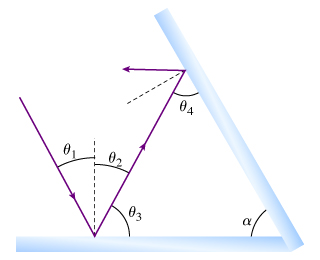# Problem: Now, find the angle θ4 shown in the figure in terms of θ1 and α.Express your answer in degrees in terms of θ1 and α.

###### FREE Expert Solution

From the law of reflection on flat surfaces:

$\overline{){{\mathbf{\theta }}}_{{\mathbf{i}}}{\mathbf{=}}{{\mathbf{\theta }}}_{{\mathbf{r}}}}$, where θi is the angle of incidence and θr is the angle of reflection.

From the figure, θi = θ1

and

θr = θ2

95% (451 ratings)###### Problem DetailsNow, find the angle θ4 shown in the figure in terms of θ1 and α.
Express your answer in degrees in terms of θ1 and α.

Frequently Asked Questions

What scientific concept do you need to know in order to solve this problem?

Our tutors have indicated that to solve this problem you will need to apply the Reflection Of Light concept. You can view video lessons to learn Reflection Of Light. Or if you need more Reflection Of Light practice, you can also practice Reflection Of Light practice problems.